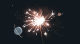# 研究科普 | 足不出户的正念心理干预方式，得劲！###### 来源公众号：正念研习（ID：zhengnianyanxi）一篇发表的在今年的元分析带来了更令人信服的结果。

① 感知压力(n = 15; g = 0.46, 95% CI [0.24, 0.68])

② 焦虑(n = 15; g = 0.28, 95% CI [0.16, 0.40])

③ 抑郁症(n = 15; g = 0.33, 95% CI [0.24, 0.43])

④ 心理幸福感(n = 5; g = 0.29, 95% CI [0.14, 0.45])

① 测试后的痛苦(n = 6; g = 0.10, 95% CI [-0.02, 0.22])

② 总体幸福感(n = 5; g = 0.14, 95% CI [-0.02, 0.29])

——END——

###### 编辑：小鲸鱼 去冰多糖

0TA在等你的回复～

（不超过200字）